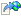﻿CommsMath Members
 CommsMath Members NetworkComms.Net Help
The CommsMath type exposes the following members.
Constructors

NameDescriptionCommsMath
Create a new empty instance of CommsMathCommsMath(List Double , List Double )
Create a new empty instance of CommsMath
Methods

NameDescriptionAddValue(Double)
Add a new value to the internal listAddValue(Double, Double)
Add a new value to the internal listCalculateMean
Return the mean of the current list.CalculateMean(List Double )
Return the mean of the provided list of valuesCalculateMean(Int32)
Return the mean of the current list.CalculateMean(List Double , List Double )
Return the mean of the provided list of valuesCalculateStdDeviation
Return the standard deviation of the current list.CalculateStdDeviation(List Double )
Return the standard deviation of the provided list of valuesCalculateStdDeviation(Int32)
Return the standard deviation of the current list.CalculateStdDeviation(List Double , List Double )
Return the standard deviation of the provided list of valuesClearList
Reset the value listEquals
Determines whether the specifiedObject is equal to the currentObject.
(Inherited fromObject.)Finalize
Allows an object to try to free resources and perform other cleanup operations before it is reclaimed by garbage collection.
(Inherited fromObject.)GetHashCode
Serves as a hash function for a particular type.
(Inherited fromObject.)GetType
Gets theType of the current instance.
(Inherited fromObject.)MemberwiseClone
Creates a shallow copy of the currentObject.
(Inherited fromObject.)ToString
Returns a string that represents the current object.
(Inherited fromObject.)TrimList
Trims the list to the provided maxCount. The most recently added items are preserved.Count